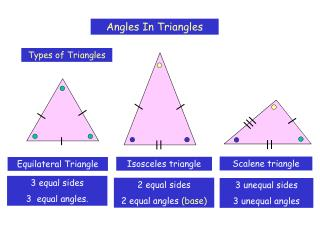DownloadDownload PresentationAngles In Triangles

# Angles In Triangles

Download Presentation## Angles In Triangles

- - - - - - - - - - - - - - - - - - - - - - - - - - - E N D - - - - - - - - - - - - - - - - - - - - - - - - - - -
##### Presentation Transcript

1. Types of Triangles Scalene triangle Isosceles triangle Equilateral Triangle 3 equal sides 3 equal angles. 2 equal sides 2 equal angles (base) 3 unequal sides 3 unequal angles Angles In Triangles

2. Any triangle containing a 90o angle is a right-angled triangle An isosceles or a scalene triangle may contain a right angle. Right-angled isosceles triangles. Right-angled scalene triangle.

3. To determine the angle sum of any Triangle 3 1 2 Angles on a straight line add to 180o How can we use this to help us? Take 3 indentical copies of this triangle like so: These are the same angles as in the triangle! The angle sum of a triangle = 1800

4. Example 1 65o Calculate angle a. a Example 2 b Calculate angles a, b and c a c Calculating unknown Angles Angle a = 180 – (90 + 65) = 180 – 155 = 25o Since the triangle is equilateral, angles a, b and c are all 60o (180/3)

5. Example 3 b Calculate angle a. a 65o Example 4 130o y Calculate angles x and y x Calculating unknown Angles Angle a = 65o (base angles of an isosceles triangle are equal). Angle b = 180 –(65 + 65) = 180 – 130 = 50o

6. Example 5 Calculate angles a and b. a b Example 6 27o Calculate angle a a 15o Calculating unknown Angles Angle a = 180 – (15 + 27) = 180 – 42 = 138o